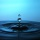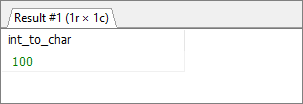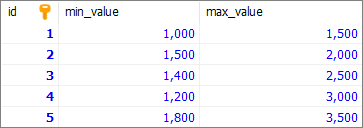Home
IT Knowledge
Inspiration
Languages
EN

# MS SQL Server - convert value to CHAR (using CONVERT() function)

0 points
Created by:Mark-Rotteveel
327

In this article, we would like to show you how to convert a value to `CHAR` type using `CONVERT()` function in MS SQL Server.

Quick solution:

``SELECT CONVERT(CHAR, value);``

## Simple example

In this example, we will convert `INT` value to `CHAR` type.

Query:

``SELECT CONVERT(CHAR, 100) AS 'int_to_char';``

Result:MS SQL Server - convert the value to char - results

## Practical example

To show how to convert the value to CHAR, we will use the following `values` table:MS SQL Server - example data used to convert int value to char with CONVERT() function

Note:

At the end of this article you can find database preparation SQL queries.

### Example

In this example, we will convert `max_value` column (which is `INT` type) from `values` table to `CHAR` type.

Query:

``````SELECT
[id], [min_value], [max_value],
CONVERT(CHAR, [max_value]) AS 'max_value_char'
FROM [values];``````

Output:

## Database preparation

`create_tables.sql` file:

``````CREATE TABLE [values] (
[id] INT IDENTITY(1,1),
[min_value] INT,
[max_value] INT,
PRIMARY KEY ([id])
);``````

`insert_data.sql` file:

``````INSERT INTO [values]
([min_value], [max_value])
values
(1000, 1500),
(1500, 2000),
(1400, 2500),
(1200, 3000),
(1800, 3500);``````